# K Dimensional Tree | Set 1 (Search and Insert)

• Difficulty Level : Medium
• Last Updated : 26 Jul, 2022

A K-D Tree(also called as K-Dimensional Tree) is a binary search tree where data in each node is a K-Dimensional point in space. In short, it is a space partitioning(details below) data structure for organizing points in a K-Dimensional space.

A non-leaf node in K-D tree divides the space into two parts, called as half-spaces.Points to the left of this space are represented by the left subtree of that node and points to the right of the space are represented by the right subtree. We will soon be explaining the concept on how the space is divided and tree is formed.

For the sake of simplicity, let us understand a 2-D Tree with an example.

The root would have an x-aligned plane, the root’s children would both have y-aligned planes, the root’s grandchildren would all have x-aligned planes, and the root’s great-grandchildren would all have y-aligned planes and so on.

Generalization:
Let us number the planes as 0, 1, 2, …(K – 1). From the above example, it is quite clear that a point (node) at depth D will have A aligned plane where A is calculated as:

A = D mod K

How to determine if a point will lie in the left subtree or in right subtree?

If the root node is aligned in planeA, then the left subtree will contain all points whose coordinates in that plane are smaller than that of root node. Similarly, the right subtree will contain all points whose coordinates in that plane are greater-equal to that of root node.

Creation of a 2-D Tree:
Consider following points in a 2-D plane:
(3, 6), (17, 15), (13, 15), (6, 12), (9, 1), (2, 7), (10, 19)

1. Insert (3, 6): Since tree is empty, make it the root node.
2. Insert (17, 15): Compare it with root node point. Since root node is X-aligned, the X-coordinate value will be compared to determine if it lies in the right subtree or in the left subtree. This point will be Y-aligned.
3. Insert (13, 15): X-value of this point is greater than X-value of point in root node. So, this will lie in the right subtree of (3, 6). Again Compare Y-value of this point with the Y-value of point (17, 15) (Why?). Since, they are equal, this point will lie in the right subtree of (17, 15). This point will be X-aligned.
4. Insert (6, 12): X-value of this point is greater than X-value of point in root node. So, this will lie in the right subtree of (3, 6). Again Compare Y-value of this point with the Y-value of point (17, 15) (Why?). Since, 12 < 15, this point will lie in the left subtree of (17, 15). This point will be X-aligned.
5. Insert (9, 1):Similarly, this point will lie in the right of (6, 12).
6. Insert (2, 7):Similarly, this point will lie in the left of (3, 6).
7. Insert (10, 19): Similarly, this point will lie in the left of (13, 15).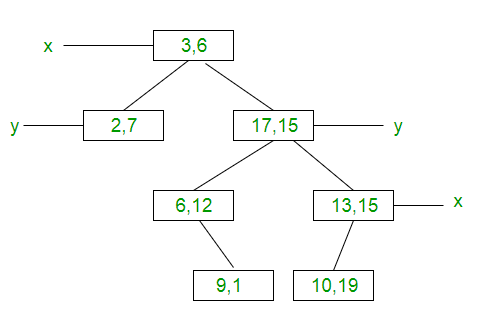How is space partitioned?
All 7 points will be plotted in the X-Y plane as follows:

1. Point (3, 6) will divide the space into two parts: Draw line X = 3.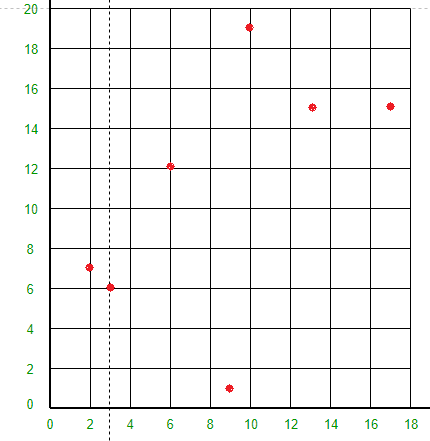2. Point (2, 7) will divide the space to the left of line X = 3 into two parts horizontally.
Draw line Y = 7 to the left of line X = 3.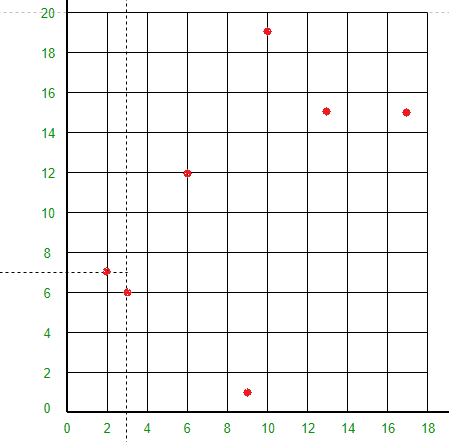3. Point (17, 15) will divide the space to the right of line X = 3 into two parts horizontally.
Draw line Y = 15 to the right of line X = 3.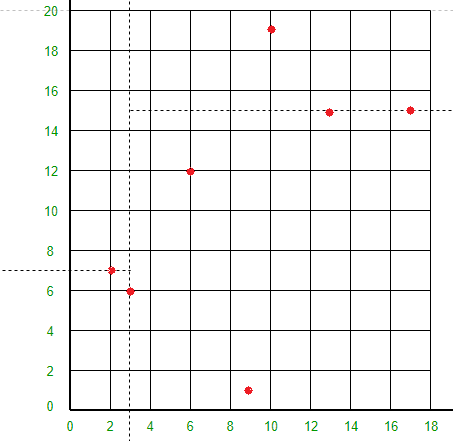• Point (6, 12) will divide the space below line Y = 15 and to the right of line X = 3 into two parts.
Draw line X = 6 to the right of line X = 3 and below line Y = 15.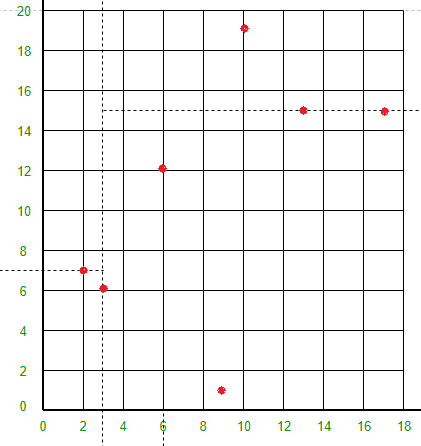• Point (13, 15) will divide the space below line Y = 15 and to the right of line X = 6 into two parts.
Draw line X = 13 to the right of line X = 6 and below line Y = 15.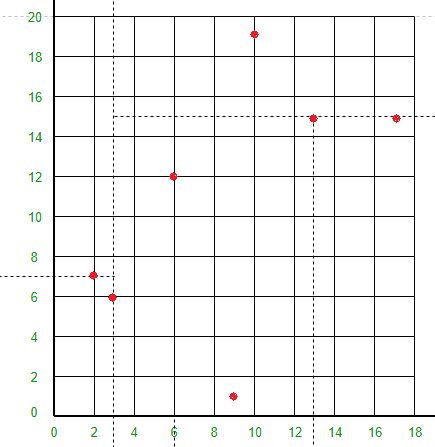• Point (9, 1) will divide the space between lines X = 3, X = 6 and Y = 15 into two parts.
Draw line Y = 1 between lines X = 3 and X = 13.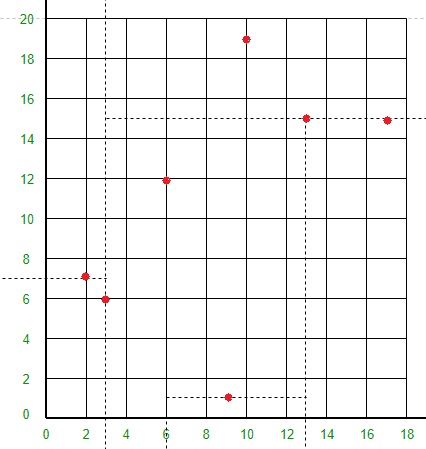• Point (10, 19) will divide the space to the right of line X = 3 and above line Y = 15 into two parts.
Draw line Y = 19 to the right of line X = 3 and above line Y = 15.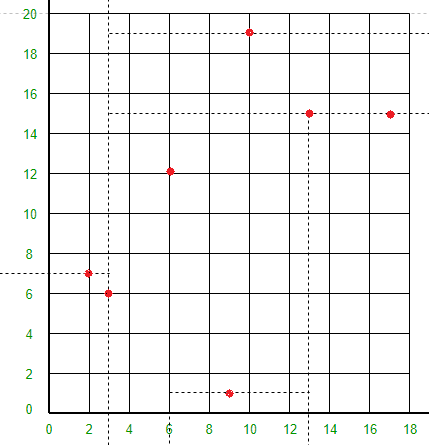Following is C++ implementation of KD Tree basic operations like search, insert and delete.

 `// A C++ program to demonstrate operations of KD tree ` `#include ` `using` `namespace` `std; ` ` `  `const` `int` `k = 2; ` ` `  `// A structure to represent node of kd tree ` `struct` `Node ` `{ ` `    ``int` `point[k]; ``// To store k dimensional point ` `    ``Node *left, *right; ` `}; ` ` `  `// A method to create a node of K D tree ` `struct` `Node* newNode(``int` `arr[]) ` `{ ` `    ``struct` `Node* temp = ``new` `Node; ` ` `  `    ``for` `(``int` `i=0; ipoint[i] = arr[i]; ` ` `  `    ``temp->left = temp->right = NULL; ` `    ``return` `temp; ` `} ` ` `  `// Inserts a new node and returns root of modified tree ` `// The parameter depth is used to decide axis of comparison ` `Node *insertRec(Node *root, ``int` `point[], unsigned depth) ` `{ ` `    ``// Tree is empty? ` `    ``if` `(root == NULL) ` `       ``return` `newNode(point); ` ` `  `    ``// Calculate current dimension (cd) of comparison ` `    ``unsigned cd = depth % k; ` ` `  `    ``// Compare the new point with root on current dimension 'cd' ` `    ``// and decide the left or right subtree ` `    ``if` `(point[cd] < (root->point[cd])) ` `        ``root->left  = insertRec(root->left, point, depth + 1); ` `    ``else` `        ``root->right = insertRec(root->right, point, depth + 1); ` ` `  `    ``return` `root; ` `} ` ` `  `// Function to insert a new point with given point in ` `// KD Tree and return new root. It mainly uses above recursive ` `// function "insertRec()" ` `Node* insert(Node *root, ``int` `point[]) ` `{ ` `    ``return` `insertRec(root, point, 0); ` `} ` ` `  `// A utility method to determine if two Points are same ` `// in K Dimensional space ` `bool` `arePointsSame(``int` `point1[], ``int` `point2[]) ` `{ ` `    ``// Compare individual pointinate values ` `    ``for` `(``int` `i = 0; i < k; ++i) ` `        ``if` `(point1[i] != point2[i]) ` `            ``return` `false``; ` ` `  `    ``return` `true``; ` `} ` ` `  `// Searches a Point represented by "point[]" in the K D tree. ` `// The parameter depth is used to determine current axis. ` `bool` `searchRec(Node* root, ``int` `point[], unsigned depth) ` `{ ` `    ``// Base cases ` `    ``if` `(root == NULL) ` `        ``return` `false``; ` `    ``if` `(arePointsSame(root->point, point)) ` `        ``return` `true``; ` ` `  `    ``// Current dimension is computed using current depth and total ` `    ``// dimensions (k) ` `    ``unsigned cd = depth % k; ` ` `  `    ``// Compare point with root with respect to cd (Current dimension) ` `    ``if` `(point[cd] < root->point[cd]) ` `        ``return` `searchRec(root->left, point, depth + 1); ` ` `  `    ``return` `searchRec(root->right, point, depth + 1); ` `} ` ` `  `// Searches a Point in the K D tree. It mainly uses ` `// searchRec() ` `bool` `search(Node* root, ``int` `point[]) ` `{ ` `    ``// Pass current depth as 0 ` `    ``return` `searchRec(root, point, 0); ` `} ` ` `  `// Driver program to test above functions ` `int` `main() ` `{ ` `    ``struct` `Node *root = NULL; ` `    ``int` `points[][k] = {{3, 6}, {17, 15}, {13, 15}, {6, 12}, ` `                       ``{9, 1}, {2, 7}, {10, 19}}; ` ` `  `    ``int` `n = ``sizeof``(points)/``sizeof``(points); ` ` `  `    ``for` `(``int` `i=0; i

Output:

```Found